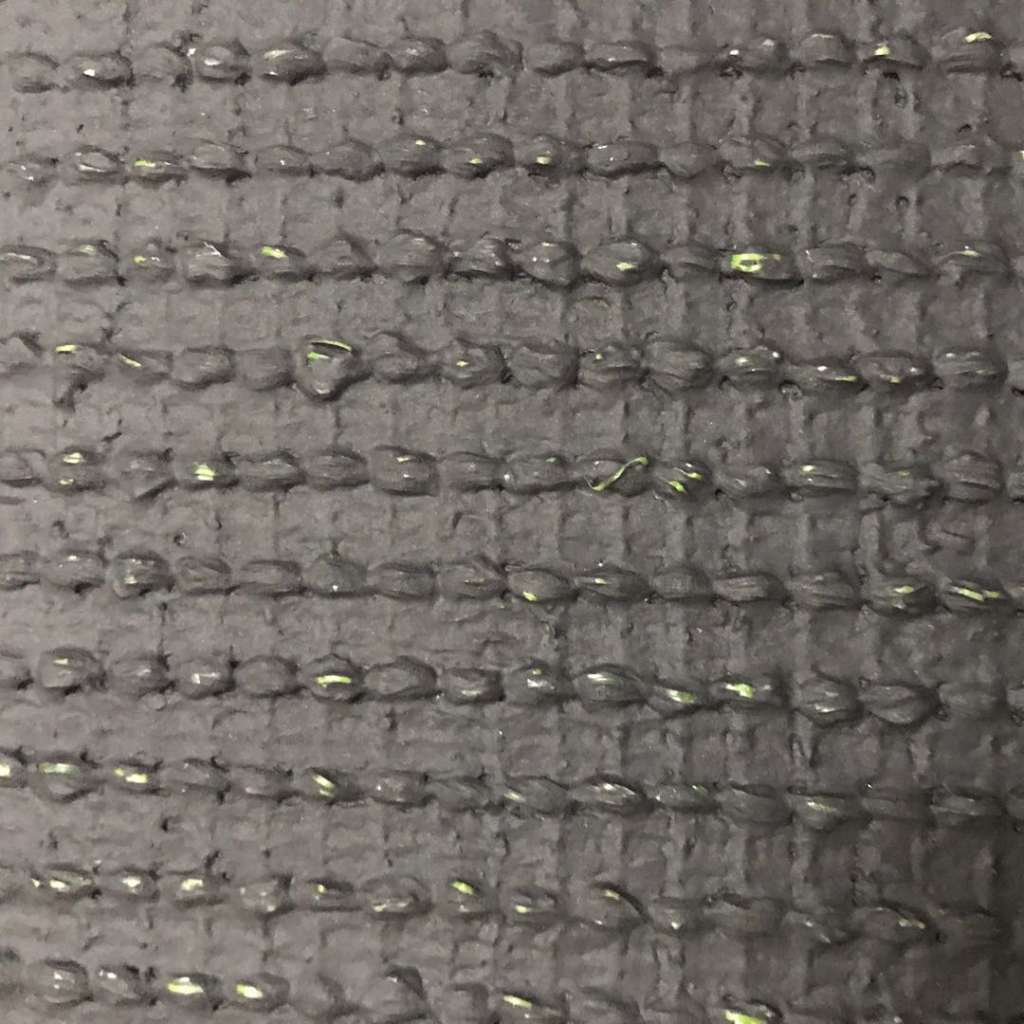# How to calculate the density of artificial grass?

Issuing time:2020-02-24 15:12Author:vitagrass

Number of stitches in artificial turf:The number of needles in artificial turf refers to the number of clusters of grass and silk per square meter of artificial turf. The number of lawn needles commonly used in football fields is 10,500 stitches, which means that there are 10,500 tufts of grass per square meter.

So how do we quickly calculate the number of clusters per square meter? If the pitch of the lawn model is 3/4, then there are 52.5 rows of grass per meter wide, and the number of clusters per meter in the longitudinal direction is 10500/52.5=200 clusters, then the number of clusters per 10cm is 20 clusters. . That is to say, in the lawn with a pitch of 3/4, the number of clusters of 10 cm in the longitudinal direction of each row is 20 clusters, which is equivalent to 10,500 needles per square meter.

The same method can be used to calculate a standard pitch of 5/8, with a lawn of 10,500 needles per square meter, and the number of clusters per 10 cm is 16.7 needles.

When the artificial turf generally refers to the parameters, it refers to the type of the lawn, the height of the grass and the number of stitches. Take a 25mm high and 16-pin lawn of our lawn. How do you calculate these values? I will tell you today how to calculate the turf parameters.

Artificial turf cluster density:

We can look at the back of the lawn according to the sample. Each cluster is called a needle. “Cluster Density” refers to how many needles per square meter, usually 16,800 needles per square meter, 18,900 needles per square meter, 22050 needles per square meter, and of course other densities, but these three are more common. .

How is the "cluster density" calculated? We all know that the area of a rectangle is long and multiplied by width, so the "cluster density" is calculated in the same way. Density conversion formula of artificial turf: number of artificial turf stitches X artificial turf stitch length. The density of artificial grass per square meter can be calculated.

Artificial turf stitch length:

The length of the "cluster density" is called the "number of needles", that is, the amount of the ruler is 10cm, and then how many needles are inside the 10cm. The common ones are 16, 18, and 21 needles.

The width of the "cluster density" is called "needle distance". Since the lawn production machine is imported from abroad, the unit is inches.

Artificial turf quality standards:

The quality standards of artificial turf are mainly determined by materials, density and gum. The following is a description of the meaning of each technical parameter and how to determine the technical parameters of the lawn based on the sample. After understanding the meaning of each technical parameter, you can judge the specification parameters of the sample yourself without labeling.

Artificial turf backing:

Artificial turf backing, the commonly used backing is the composite bottom & mesh bottom. The difference is that a layer of mesh cloth is added to the bottom of the grid, which is mainly used to strengthen the grass.

Share to：
About VITA We are the manufacturer of artificial grass for sports, landscaping, golf and etc. Best quality prodct with our one-stop service and happy price help us win lots of repeated customers.粤公网安备 44011102002606号